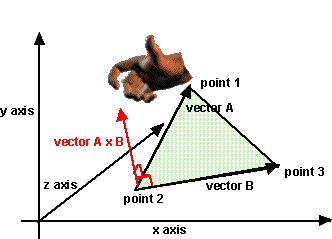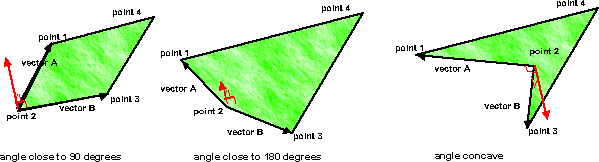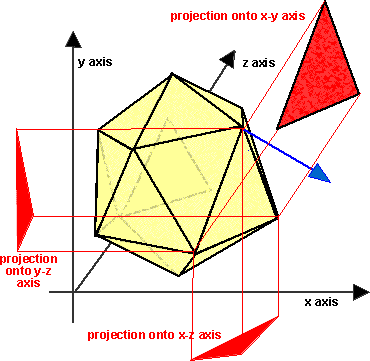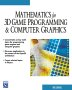# Maths - Normals

A normal is a unit vector which is perpendicular to the surface. It is often used in rendering 3D scenes to calculate now the light sources are reflected to the camera view. So the normals are often included in the scene graph to speed up rendering. For more about using unit length vectors to specify direction see here.

Note: In OpenGL etc. normals are only used for lighting, back face culling is done using the winding order of the coordinates.

## Calculation of normals from mesh data

The normals can be calculated from Indexed Face Set coordinates as follows:

1. Calculate the normal to each face.
2. If you require a normal per vertex (without crease angle), calculate this by adding the normals for each face adjoining the vertex, and then normalising.

## Calculate the normal to each face.

### Conventions• The vector points outward from the object, i.e. the object will be seen from the side the vector points toward.
• The points are assumed to be ordered the same way round for all faces (known as the winding order) which allows the normal direction to be determined.
• This order is given by the right hand rule, i.e. if the fingers of the right hand curl in the direction given by point1, point2, point3... then the thumb will point in the direction of the normal.
• The conventions for the coordinates are shown here.

Method 1 - Using cross product.This can be calculated by taking the cross product of any 2 of its edges, and then normalising. We need to be careful to check the following conditions:

1. The two edges chosen must not be parallel, i.e. the angle between the edges must not be 0 or 180 degrees. The normal will be more accurate if the angle between the lines is closer to 90 degrees.
2. The length of the edges must be non-zero and the normal will be more accurate if the length is high compared with the accuracy of the coordinates.
3. If the angle is concave then the direction of the normal needs to be reversed.These conditions are more likely to be met if the edges chosen are adjacent to each other, i.e. if they share a common point then they are less likely to be parallel to each other.

In order to avoid a concave angle, then this common point should be further away from the centre than the other points.

Java code to generate a normal to the whole face:

```sfvec3f generateFaceNormal(sfvec3f a,sfvec3f b,sfvec3f c){
sfvec3f v1 = sfvec3f.sub(a,b);
sfvec3f v2 = sfvec3f.sub(a,c);
v1.cross(v2);
v1.normalize();
return v1;
}```

Managed C++code to generate a normal to the whole face:

```sfvec3f* triangleHolder::generateFaceNormal(sfvec3f* a,sfvec3f* b,sfvec3f* c){
sfvec3f* v1 = sfvec3f::sub(a,b);
sfvec3f* v2 = sfvec3f::sub(a,c);
v1->cross(v2);
v1->normalize();
return v1;
}```

Method 2 - using the area projections.

If the above conditions are likely to cause problems then there is a more complex method which uses all the points:Calculate the area of the polygon when projected onto the y-z, x-z and x-y axes.

This is equivalent to a sum of triangle normal vectors formed by all edges of the polygon with respect to some reference point in the plane such as the centroid of all vertices.

## Per vertex Normal

We can now calculate this by adding the normals for each face adjoining the vertex, and then normalising.

## creaseAngle

The creaseAngle field, used by the ElevationGrid, Extrusion, and IndexedFaceSet nodes, affects how default normals are generated. If the angle between the geometric normals of two adjacent faces is less than the crease angle, normals shall be calculated so that the faces are smooth-shaded across the edge; otherwise, normals shall be calculated so that a lighting discontinuity across the edge is produced. For example, a crease angle of 0.5 radians means that an edge between two adjacent polygonal faces will be smooth shaded if the geometric normals of the two faces form an angle that is less than 0.5 radians. Otherwise, the faces will appear faceted. Crease angles shall be greater than or equal to 0.0.

### Managed C++ code

I don't claim this code is very good so please send me improvements.

```void triangleHolder::generateNormals(double creaseAngle){
if (normals) normals->clear();
else normals = new mfvec3f();
int vertexPerFace =3;
for (int i=0;i<coordinates->howmany();i=i+vertexPerFace) {
sfvec3f* a=coordinates->getEntry(i);
sfvec3f* b=coordinates->getEntry(i+1);
sfvec3f* c=coordinates->getEntry(i+2);
sfvec3f* sfv=generateFaceNormal(a,b,c);
normals->setEntry(sfv,i);
normals->setEntry(sfv,i+1);
normals->setEntry(sfv,i+2);
if (vertexPerFace>3) normals->setEntry(sfv,i+3);
}
if (creaseAngle < 0.9) { // average out normals for identical points
mfint32* coordInd = new mfint32(); // build an array which shows the first index
// where a coordinate occurs
for (int i=0;i<coordinates->howmany();i++) {
sfvec3f* a=coordinates->getEntry(i);
int index = coordinates->findIndex(a);
if (index < 0) index = i;
}
for (int j=0;j<coordInd->howmany();j++) {
int k=coordInd->getValue(j);
if (k==j) { // this is the first occurance of this coordinate
// so we need to find the others and average out their normals
// find number of coordinates with this value
int n=1;
for (int m=k+1;m<coordInd->howmany();m++) {
if (k==coordInd->getValue(m)) n++;
}
if (n>1) { // if there are more than 1 average out their normals
sfvec3f* averagedNorm=new sfvec3f(normals->getEntry(k));
for (int m=k+1;m<coordInd->howmany();m++) {
if (k==coordInd->getValue(m)) {
}
}
averagedNorm->normalize();
for (int m=0;m<coordInd->howmany();m++) { // put avaraged values back in norms
if (k==coordInd->getValue(m)) {
normals->setEntry(averagedNorm,m);
}
}
}
}
}
}```

 metadata block see also: tutorial Correspondence about this page Book Shop - Further reading. Where I can, I have put links to Amazon for books that are relevant to the subject, click on the appropriate country flag to get more details of the book or to buy it from them.Mathematics for 3D game Programming - Includes introduction to Vectors, Matrices, Transforms and Trigonometry. (But no euler angles or quaternions). Also includes ray tracing and some linear & rotational physics also collision detection (but not collision response).Covers VRML 2 (but not the upcoming X3D standard). A good introduction to all VRML2 node types and how to build them. Other Math Books Specific to this page here:

This site may have errors. Don't use for critical systems.